Courses

# Modes of Heat Transfer - MCQ Test 2

## 30 Questions MCQ Test RRB JE for Mechanical Engineering | Modes of Heat Transfer - MCQ Test 2

Description
This mock test of Modes of Heat Transfer - MCQ Test 2 for Mechanical Engineering helps you for every Mechanical Engineering entrance exam. This contains 30 Multiple Choice Questions for Mechanical Engineering Modes of Heat Transfer - MCQ Test 2 (mcq) to study with solutions a complete question bank. The solved questions answers in this Modes of Heat Transfer - MCQ Test 2 quiz give you a good mix of easy questions and tough questions. Mechanical Engineering students definitely take this Modes of Heat Transfer - MCQ Test 2 exercise for a better result in the exam. You can find other Modes of Heat Transfer - MCQ Test 2 extra questions, long questions & short questions for Mechanical Engineering on EduRev as well by searching above.
QUESTION: 1

### A steel ball of mass 1kg and specific heat 0.4 kJ/kg is at a temperature of 60°C. It is dropped into 1kg water at 20°C. The final steady state temperature of water is:

Solution:

1 kg steel ball and 1 kg water are both having finite heat capacity

Let the final temperature (at which thermal equilibrium is attained) be T

Heat lost by steel ball = Heat gained by water

1 (kg) x 0.4 (kJ/kg-°C) x (60 – T) (°C) = 1 (kg) x 4.18 (kJ/kg-°C) x (T – 20)

T = 23.5°C

QUESTION: 2

### A large concrete slab 1 m thick has one dimensional temperature distribution:   T = 4 – 10x + 20x2 + 10x3 Where T is temperature and x is distance from one face towards other face of wall. If the slab material has thermal diffusivity of 2 × 10-3 m2/hr,what is the rate of change of temperature at the other face of the wall?

Solution:

Ans. (b)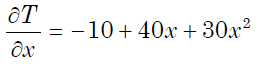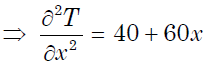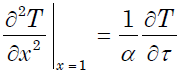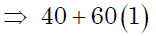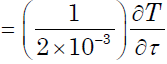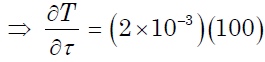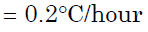QUESTION: 3

### In descending order of magnitude, the thermal conductivity of a. Pure iron,                                                                             b. Liquid water, c. Saturated water vapour, and d. Pure aluminium can be arranged as

Solution:
QUESTION: 4

Thermal diffusivity of a substance is:

Solution:

Ans. (b) Thermal diffusivity

(α) =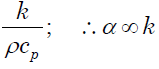QUESTION: 5

Match List-I and List-II and select the correct answer using the codes given below the lists:

hm - mass transfer coefficient,
D - molecular diffusion coefficient,
L - characteristic length dimension,
k - thermal conductivity,
ρ - density,
Cp - specific heat at constant pressure, μ- dynamic viscosity)
List-I                                                                             List-II
A. Schmidt number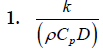B. Thermal diffusivity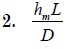C. Lewis number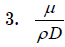D. Sherwood number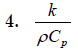Codes:    A B C D         A B C D
(a)          4 3 2 1       (b) 4 3 1 2
(c)          3 4 2 1       (d) 3 4 1 2

Solution:

Ans. (d)

QUESTION: 6

A furnace is made of a red brick wall of thickness 0.5 m and conductivity 0.7 W/mK. For the same heat loss and temperature drop,this can be replaced by a layer of diatomite earth of conductivity 0.14W/mK and thickness

Solution:

Ans. (b) For thick place homogeneous wall, heat loss =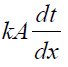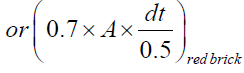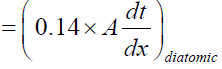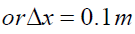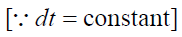QUESTION: 7

In which one of the following materials, is the heat energy propagation minimum due to conduction heat transfer?

Solution:

Ans. (d) Heat energy propagation minimum due to conduction heat transfer in case of Air as its thermal conductivity is low.

QUESTION: 8

Assertion (A): The leakage heat transfer from the outside surface of asteel pipe carrying hot gases is reduced to a greater extent on providing refractory brick lining on the inside of the pipe as compared to that with brick lining on the outside.
Reason (R): The refractory brick lining on the inside of the pipe offersa higher thermal resistance.

Solution:

Ans. (a)

QUESTION: 9

In MLT θ system (T being time and θ temperature), what is the dimension of thermal conductivity?

Solution:

Ans. (c)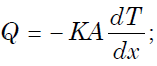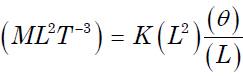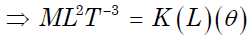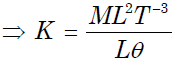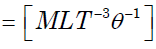QUESTION: 10

Assertion (A): Non-metals are having higher thermal conductivity than metals. Reason (R): Free electrons In the metals are higher than those of nonmetals.

Solution:

Ans. (d) Non-metals have lower thermal conductivity and free electrons in metal higher then non metal therefore (d) is the answer.

QUESTION: 11

In a case of one dimensional heat conduction in a medium with constant properties, T is the temperature at position x, at time t. Then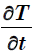is proportional to:

Solution:

Ans. (d) One dimensional, Unsteady state, without internal heat generation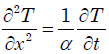QUESTION: 12

A building has to be maintained at 21°C (dry bulb) and 14.5°C. The outside temperature is –23°C (dry bulb) and the internal and external surface heat transfer coefficients are 8 W/m2K and 23 W/m2K respectively. If the building wall has a thermal conductivity of 1.2W/mK, the minimum thickness (in m) of the wall required to prevent condensation is:

Solution:
QUESTION: 13

Consider steady-state heat conduction across the thickness in a plane composite wall (as shown in the figure) exposed to convection conditions on both sides.
Given: hi = 20 W/m2K; ho = 50 W/m2K; T∞.i = 20° C ; T∞.i = -  2° C ;
k1 = 20 W/mK; k2 = 50 W/mK; L= 0.30 m and L2 = 0.15 m.
Assuming negligible contact resistance between the wall surfaces, the interface temperature, T (in °C), of the two walls will be: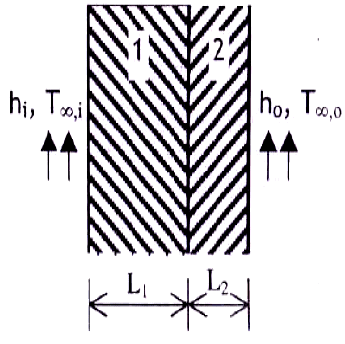Solution:

Ans. (c)
Q =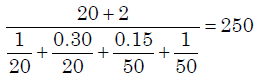or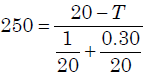or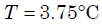QUESTION: 14

The temperature variationunder steady heat conductionacross a composite slab of twomaterials with thermalconductivities K1 and K2 isshown in figure. Then, whichone of the followingstatements holds?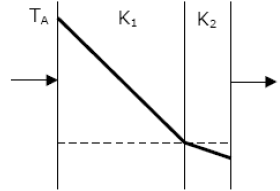Solution:

Ans. (d) Lower the thermal conductivity greater will be the slope of the temperature distribution curve (The curve shown here is temperature distribution curve).

QUESTION: 15

A steel steam pipe 10 cm inner diameter and 11 cm outer diameter iscovered with insulation having the thermal conductivity of 1 W/mK. Ifthe convective heat transfer coefficient between the surface ofinsulation and the surrounding air is 8 W / m2K, then critical radius ofinsulation is:

Solution:

Ans. (c) Critical radius of insulation (rc) = k/h= 1/8 m = 12.5cm

QUESTION: 16

Two insulating materials of thermal conductivity K and 2K are available for lagging a pipe carrying a hot fluid. If the radial thickness of each material is the same.

Solution:
QUESTION: 17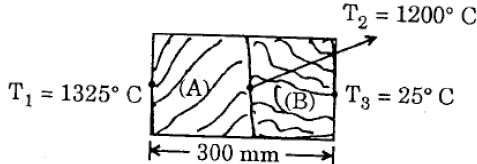A wall as shown above is made up of two layers (A) and (B). The temperatures are also shown in the sketch. The ratio of thermal conductivity of two layers is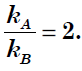What is the ratio of thickness of two layers?

Solution:

Ans. (b)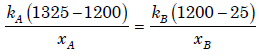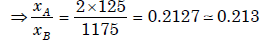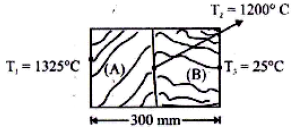QUESTION: 18

A 0.5 m thick plane wall has its two surfaces kept at 300°C and 200°C.Thermal conductivity of the wall varies linearly with temperature and its values at 300°C and 200°C are 25 W/mK and 15W/mK respectively. Then the steady heat flux through the wall is:

Solution:

Ans. (c)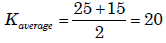[As it is varying linearly]

QUESTION: 19

Heat is conducted through a 10 cm thick wall at the rate of 30 W/m2 when the temperature difference across the wall is 10oC. What is the thermal conductivity of the wall?

Solution:

Ans. (b)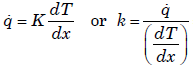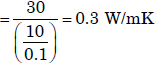QUESTION: 20

6.0 kJ of conduction heat transfer has to take place in 10 minutes from one end to other end of a metallic cylinder of 10 cm2 cross-sectional area, length 1 meter and thermal conductivity as 100 W/mK. What is the temperature difference between the two ends of the cylindrical bar?

Solution:

Ans. (b)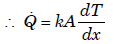or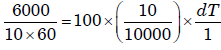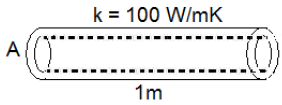or dt = 1000C

QUESTION: 21

A composite hollow sphere with steady internal heating is made of 2layers of materials of equal thickness with thermal conductivities inthe ratio of 1 : 2 for inner to outer layers. Ratio of inside to outside diameter is 0.8. What is ratio of temperature drop across the inner andouter layers?

Solution:

Ans. (d)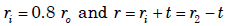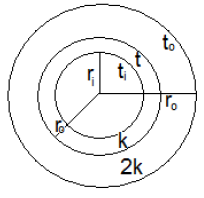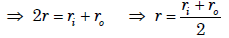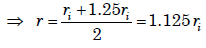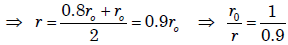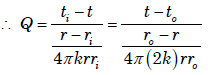QUESTION: 22

The heat flow through a composite cylinder is given by the equation:
(symbols have the usual meaning)

Solution:

Ans. (a)

QUESTION: 23

A solid sphere and a hollow sphere of the same material and size are heated to the same temperature and allowed to cool in the same surroundings. If the temperature difference between the body and thatof the surroundings is T, then

Solution:

Ans. (c)

QUESTION: 24

The outer surface of a long cylinder is maintained at constant temperature. The cylinder does not have any heat source.
The temperature in the cylinder will:

Solution:

Ans. (d)

QUESTION: 25

What will be the geometric radius of heat transfer for a hollow sphere of inner and outer radii r1 and r?

Solution:

Ans. (a)

QUESTION: 26

The overall heat transfer coefficient U for a plane composite wall of n layers is given by (the thickness of the ith layer is ti, thermal conductivity of the it h layer is ki, convective heat transfer co-efficient is h)

Solution:

Ans. (a)

QUESTION: 27

The heat flow equation through a cylinder of inner radius “r1” and outer radius “r2” is desired in the same form as that for heat flow through a plane wall. The equivalent area Am is given by:

Solution:

Ans. (d)

QUESTION: 28

A flat plate has thickness 5 cm, thermal conductivity 1 W/(mK),convective heat transfer coefficients on its two flat faces of 10 W/(m2K)and 20 W/(m2K). The overall heat transfer co-efficient for such a flatplate is:

Solution:
QUESTION: 29

A steel plate of thickness 5 cm and thermal conductivity 20W/mK is subjected to auniform heat flux of 800 W/mon one surface 'A' and transfers heat by convection with a heat transfer coefficientof 80 W/m2K from the other surface 'B' into ambientair Tα of 25°C. The temperature of the surface 'B'transferring heat by convection is:

Solution:

Ans. (b)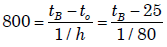QUESTION: 30

A composite slab has two layers of different materials having internal conductivities k1 and k2. If each layer has the same thickness, then what is the equivalent thermal conductivity of the slab?

Solution:

Ans. (d) Same questions [IES-1997] and [IES-2000]